# matplotlib.gridspec.GridSpec#

class matplotlib.gridspec.GridSpec(nrows, ncols, figure=None, left=None, bottom=None, right=None, top=None, wspace=None, hspace=None, width_ratios=None, height_ratios=None)[source]#

Bases: `GridSpecBase`

A grid layout to place subplots within a figure.

The location of the grid cells is determined in a similar way to `SubplotParams` using left, right, top, bottom, wspace and hspace.

Indexing a GridSpec instance returns a `SubplotSpec`.

Parameters:
nrows, ncolsint

The number of rows and columns of the grid.

figure`Figure`, optional

Only used for constrained layout to create a proper layoutgrid.

left, right, top, bottomfloat, optional

Extent of the subplots as a fraction of figure width or height. Left cannot be larger than right, and bottom cannot be larger than top. If not given, the values will be inferred from a figure or rcParams at draw time. See also `GridSpec.get_subplot_params`.

wspacefloat, optional

The amount of width reserved for space between subplots, expressed as a fraction of the average axis width. If not given, the values will be inferred from a figure or rcParams when necessary. See also `GridSpec.get_subplot_params`.

hspacefloat, optional

The amount of height reserved for space between subplots, expressed as a fraction of the average axis height. If not given, the values will be inferred from a figure or rcParams when necessary. See also `GridSpec.get_subplot_params`.

width_ratiosarray-like of length ncols, optional

Defines the relative widths of the columns. Each column gets a relative width of `width_ratios[i] / sum(width_ratios)`. If not given, all columns will have the same width.

height_ratiosarray-like of length nrows, optional

Defines the relative heights of the rows. Each row gets a relative height of `height_ratios[i] / sum(height_ratios)`. If not given, all rows will have the same height.

get_subplot_params(figure=None)[source]#

Return the `SubplotParams` for the GridSpec.

In order of precedence the values are taken from

• non-None attributes of the GridSpec

• the provided figure

• `rcParams["figure.subplot.*"]`

locally_modified_subplot_params()[source]#

Return a list of the names of the subplot parameters explicitly set in the GridSpec.

This is a subset of the attributes of `SubplotParams`.

Adjust subplot parameters to give specified padding.

Parameters:

Padding between the figure edge and the edges of subplots, as a fraction of the font-size.

Padding (height/width) between edges of adjacent subplots. Defaults to pad.

recttuple (left, bottom, right, top), default: None

(left, bottom, right, top) rectangle in normalized figure coordinates that the whole subplots area (including labels) will fit into. Default (None) is the whole figure.

update(**kwargs)[source]#

Update the subplot parameters of the grid.

Parameters that are not explicitly given are not changed. Setting a parameter to None resets it to `rcParams["figure.subplot.*"]`.

Parameters:
left, right, top, bottomfloat or None, optional

Extent of the subplots as a fraction of figure width or height.

wspace, hspacefloat, optional

Spacing between the subplots as a fraction of the average subplot width / height.

## Examples using `matplotlib.gridspec.GridSpec`#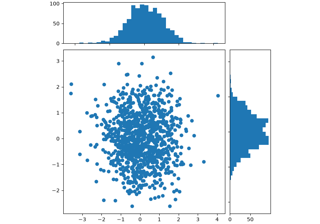Scatter plot with histograms

Scatter plot with histograms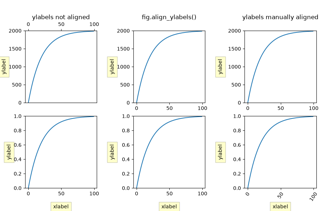Aligning Labels

Aligning Labels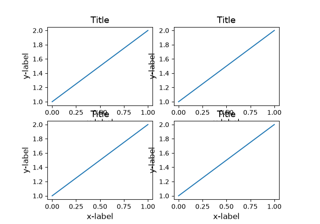Resizing axes with constrained layout

Resizing axes with constrained layout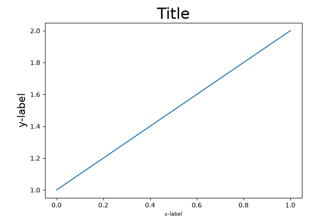Resizing axes with tight layout

Resizing axes with tight layout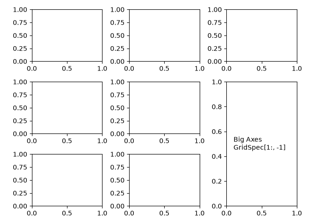Combining two subplots using subplots and GridSpec

Combining two subplots using subplots and GridSpec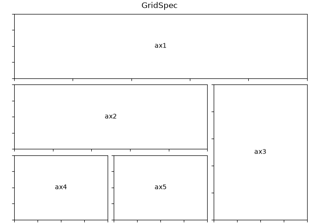Using Gridspec to make multi-column/row subplot layouts

Using Gridspec to make multi-column/row subplot layouts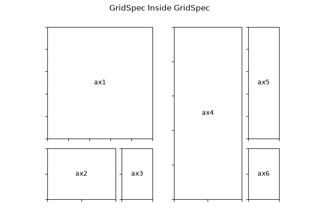Nested Gridspecs

Nested Gridspecs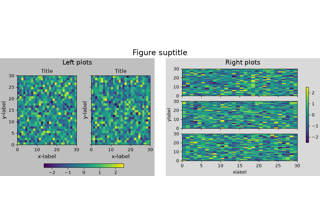Figure subfigures

Figure subfigures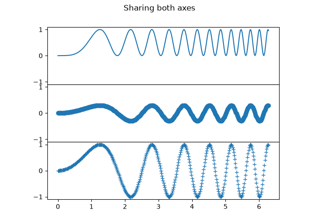Creating multiple subplots using plt.subplots

Creating multiple subplots using ``plt.subplots``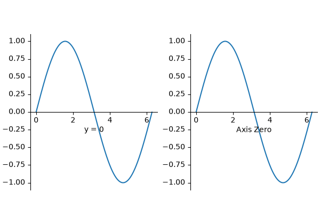Custom spines with axisartist

Custom spines with axisartistGridSpec demo

GridSpec demoNested GridSpecs

Nested GridSpecs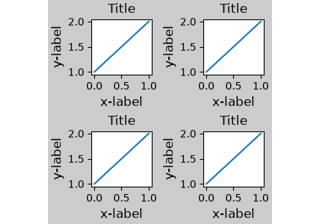Constrained Layout Guide

Constrained Layout Guide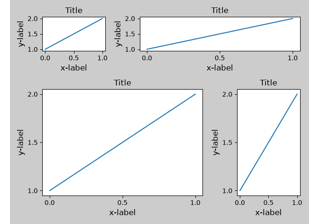Tight Layout guide

Tight Layout guide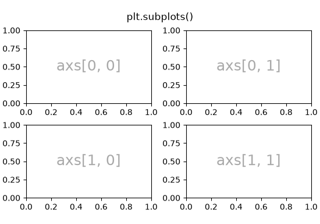Arranging multiple Axes in a Figure

Arranging multiple Axes in a Figure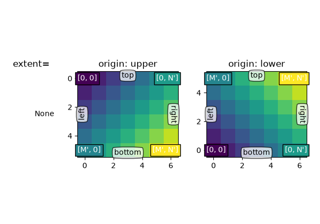origin and extent in imshow

*origin* and *extent* in `~.Axes.imshow`